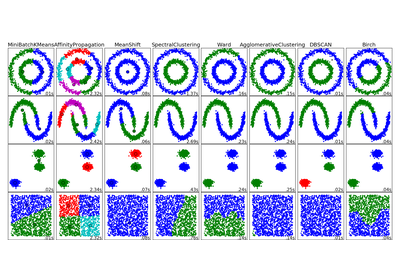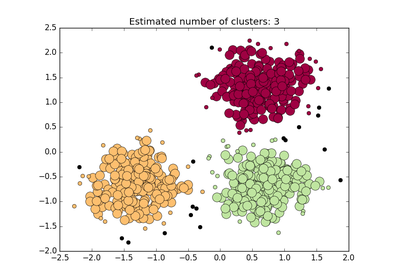# `sklearn.cluster`.DBSCAN¶

class `sklearn.cluster.``DBSCAN`(eps=0.5, min_samples=5, metric='euclidean', algorithm='auto', leaf_size=30, p=None, n_jobs=1)[source]

Perform DBSCAN clustering from vector array or distance matrix.

DBSCAN - Density-Based Spatial Clustering of Applications with Noise. Finds core samples of high density and expands clusters from them. Good for data which contains clusters of similar density.

Read more in the User Guide.

Parameters: eps : float, optional The maximum distance between two samples for them to be considered as in the same neighborhood. min_samples : int, optional The number of samples (or total weight) in a neighborhood for a point to be considered as a core point. This includes the point itself. metric : string, or callable The metric to use when calculating distance between instances in a feature array. If metric is a string or callable, it must be one of the options allowed by metrics.pairwise.calculate_distance for its metric parameter. If metric is “precomputed”, X is assumed to be a distance matrix and must be square. X may be a sparse matrix, in which case only “nonzero” elements may be considered neighbors for DBSCAN. New in version 0.17: metric precomputed to accept precomputed sparse matrix. algorithm : {‘auto’, ‘ball_tree’, ‘kd_tree’, ‘brute’}, optional The algorithm to be used by the NearestNeighbors module to compute pointwise distances and find nearest neighbors. See NearestNeighbors module documentation for details. leaf_size : int, optional (default = 30) Leaf size passed to BallTree or cKDTree. This can affect the speed of the construction and query, as well as the memory required to store the tree. The optimal value depends on the nature of the problem. p : float, optional The power of the Minkowski metric to be used to calculate distance between points. n_jobs : int, optional (default = 1) The number of parallel jobs to run. If `-1`, then the number of jobs is set to the number of CPU cores. core_sample_indices_ : array, shape = [n_core_samples] Indices of core samples. components_ : array, shape = [n_core_samples, n_features] Copy of each core sample found by training. labels_ : array, shape = [n_samples] Cluster labels for each point in the dataset given to fit(). Noisy samples are given the label -1.

Notes

See examples/cluster/plot_dbscan.py for an example.

This implementation bulk-computes all neighborhood queries, which increases the memory complexity to O(n.d) where d is the average number of neighbors, while original DBSCAN had memory complexity O(n).

Sparse neighborhoods can be precomputed using `NearestNeighbors.radius_neighbors_graph` with `mode='distance'`.

References

Ester, M., H. P. Kriegel, J. Sander, and X. Xu, “A Density-Based Algorithm for Discovering Clusters in Large Spatial Databases with Noise”. In: Proceedings of the 2nd International Conference on Knowledge Discovery and Data Mining, Portland, OR, AAAI Press, pp. 226-231. 1996

Methods

 `fit`(X[, y, sample_weight]) Perform DBSCAN clustering from features or distance matrix. `fit_predict`(X[, y, sample_weight]) Performs clustering on X and returns cluster labels. `get_params`([deep]) Get parameters for this estimator. `set_params`(\*\*params) Set the parameters of this estimator.
`__init__`(eps=0.5, min_samples=5, metric='euclidean', algorithm='auto', leaf_size=30, p=None, n_jobs=1)[source]
`fit`(X, y=None, sample_weight=None)[source]

Perform DBSCAN clustering from features or distance matrix.

Parameters: X : array or sparse (CSR) matrix of shape (n_samples, n_features), or array of shape (n_samples, n_samples) A feature array, or array of distances between samples if `metric='precomputed'`. sample_weight : array, shape (n_samples,), optional Weight of each sample, such that a sample with a weight of at least `min_samples` is by itself a core sample; a sample with negative weight may inhibit its eps-neighbor from being core. Note that weights are absolute, and default to 1.
`fit_predict`(X, y=None, sample_weight=None)[source]

Performs clustering on X and returns cluster labels.

Parameters: X : array or sparse (CSR) matrix of shape (n_samples, n_features), or array of shape (n_samples, n_samples) A feature array, or array of distances between samples if `metric='precomputed'`. sample_weight : array, shape (n_samples,), optional Weight of each sample, such that a sample with a weight of at least `min_samples` is by itself a core sample; a sample with negative weight may inhibit its eps-neighbor from being core. Note that weights are absolute, and default to 1. y : ndarray, shape (n_samples,) cluster labels
`get_params`(deep=True)[source]

Get parameters for this estimator.

Parameters: deep : boolean, optional If True, will return the parameters for this estimator and contained subobjects that are estimators. params : mapping of string to any Parameter names mapped to their values.
`set_params`(**params)[source]

Set the parameters of this estimator.

The method works on simple estimators as well as on nested objects (such as pipelines). The latter have parameters of the form `<component>__<parameter>` so that it’s possible to update each component of a nested object.

Returns: self :

## Examples using `sklearn.cluster.DBSCAN`¶Comparing different clustering algorithms on toy datasetsDemo of DBSCAN clustering algorithm